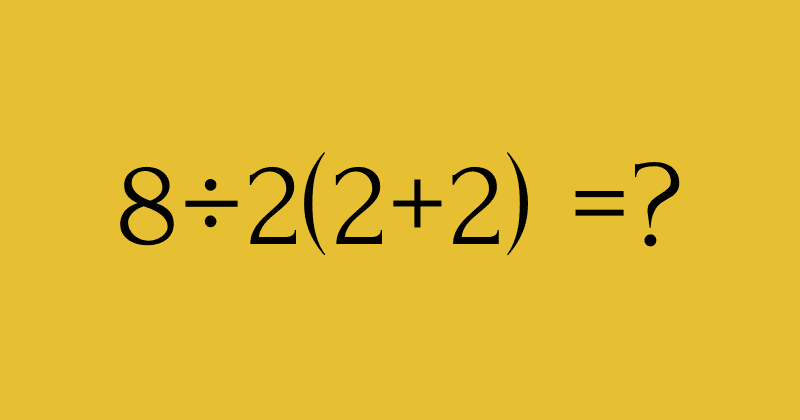Fun Puzzle: Can You Solve This Deceivingly Simple Math Equation That Left the Internet Divided?

# Fun Puzzle: Can You Solve This Deceivingly Simple Math Equation That Left the Internet Divided?

Although it appeared deceivingly simple, the equation left the internet divided and the Twitterverse baffled at the results.Not many can say that mathematics has been a piece of cake for them in school. For some, the struggle to solve equations advances to such a level that it turns into an actual fear called "math phobia." But it is mainly a feeling of anxiety or negative attitude that prevents one from tackling mathematical problems efficiently. We all know that practice makes perfect because numbers are not really the enemy now, are they?

Before you can begin crunching actual numbers, a sound knowledge of the fundamentals is absolutely necessary. Once you get a hold of the basics, you'll be able to solve sums in no time and the same goes for an equation that left everyone in a doozy after being posted on social media. Although it appeared deceivingly simple, the equation left the internet divided and the Twitterverse baffled at the results.

It all started when a user innocently asked everyone to solve this equation: "8÷2(2+2) = ?" And Twitter users erupted almost immediately as people were posting two very conflicting answers. While the problem seemed to be something that you might have learned back in fourth grade algebra, it managed to blow everyone's mind. Now go on, give it a try.

.

.

.

Not sure how to go about solving the equation?

Here's a hint:

Those who grew up in the United States would have immediately remembered the frequently used "order of operations" as "Please Excuse My Dear Aunt Sally," or P = parentheses, E is exponents, M and D are multiplication and division, and A and S are addition and subtraction. Use this to tackle the problem.

.

.

Did you arrive at 1? Or is the answer 16? Let's take a look below.

If we tackle the equation keeping the operation in mind, first we solve the parentheses or the equation within brackets. So, I first did this: 2 + 2 = 4, which wasn't that hard, was it? After this we see the equation as 8 ÷2 (4) = ? and if we follow the PEDMAS rule then we solve the parentheses yet again and multiplying 2 and 4 leaves us with 8. Now, we have 8÷8, which equals one. Voila, one is the answer!

I was very sure that most people would have gotten the same answer as me and many did. Those living in the other parts of the world like in the U.K. applied the BODMAS rule and ended up with the answer 1. But just as I was getting ready to celebrate by AP Calculus skills, I was left surprised to see just how many people had come up with a totally different answer, most of which was 16.

To arrive at this, the first step is the same where we solve the parentheses and believe that we are left with 8 ÷ 2 x 4. Citing PEMDAS or BODMAS, some claimed that the order of operations did not necessarily demand multiplication to happen first and then division. They explained how multiplication and division have equal hierarchy and that one can solve them in whichever order they appear in an equation. Based on this logic, many Twitter users solved 8 ÷ 2 x 4 like this: 8 divided by 2 is 4. 4 x 4 is 16.

I understand that the difference in answers could be a little frustrating and this was exactly what led to the virality of the sum as many came up with either 16 or 1 as the answer. As an all-out brawl broke out between the ones and the 16s, even Berkeley admitted that it was a poorly written equation and that even they were uncertain about the correct answer.

But the author of The Joy of Game Theory: An Introduction to Strategic Thinking, Presh Talwalker, addressed the controversy on his YouTube channel and said that people who got 1 as the answer were using an outdated version of the order of operations while others using the modern one. According to Insider, he added that typing the equation into a modern calculator will fetch the answer 16 and that you would have to add another set of parentheses to arrive at 1.

References:

https://www.insider.com/viral-math-problem-solution-dividing-the-internet-2019-7

https://math.berkeley.edu/~gbergman/misc/numbers/ord_ops.html